# Cracking the top Amazon coding interview questions

Jun 17, 2020 - 27 min read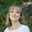Amanda FawcettLanding a job at Amazon is a dream for many developers around the globe. Amazon is one of the largest companies in the world, with a workforce of over half a million strong.

For you to join them, you’ll need to complete their unique interview that combines technical and leadership knowledge.

Today, I’ll walk you through everything you need to crack the Amazon interview, including coding questions and a step-by-step preparation guide.

Today we will go over the following:

#### Coding and Algorithms Skill Assessment

Take our short quiz on programming and system design to get personalized recommendations designed to help you ace your next interview.###### Coding and Algorithms Skill Assessment

By Educative## 45 common Amazon coding interview questions

### 1. Find the missing number in the array

You are given an array of positive numbers from 1 to n, such that all numbers from 1 to n are present except one number x. You have to find x. The input array is not sorted. Look at the below array and give it a try before checking the solution.

n = 8 missing number = 6
def find_missing(input):
#TODO: Write - Your - Code
return -1

Click here to view the solution in C++, Java, JavaScript, and Ruby.

Runtime Complexity: Linear, $O(n)$

Memory Complexity: Constant, $O(1)$

A naive solution is to simply search for every integer between 1 and n in the input array, stopping the search as soon as there is a missing number. But we can do better. Here is a linear, $O(n)$, solution that uses the arithmetic series sum formula.​​ Here are the steps to find the missing number:

• Find the sum sum_of_elements of all the numbers in the array. This would require a linear scan, $O(n)$.
• Then find the sum expected_sum of first n numbers using the arithmetic series sum formula
• The difference between these i.e. expected_sum - sum_of_elements, is the missing number in the array.

### 2. Determine if the sum of two integers is equal to the given value

Given an array of integers and a value, determine if there are any two integers in the array whose sum is equal to the given value. Return true if the sum exists and return false if it does not. Consider this array and the target sums:

def find_sum_of_two(A, val):
#TODO: Write - Your - Code
return;

Click here to view the solution in C++, Java, JavaScript, and Ruby.

Runtime Complexity: Linear, $O(n)$

Memory Complexity: Linear, $O(n)$

You can use the following algorithm to find a pair that add up to the target (say val).

• Scan the whole array once and store visited elements in a hash set.
• During scan, for every element e in the array, we check if val - e is present in the hash set i.e. val - e is already visited.
• If val - e is found in the hash set, it means there is a pair (e, val - e) in array whose sum is equal to the given val.
• If we have exhausted all elements in the array and didn’t find any such pair, the function will return false

### 3. Merge two sorted linked lists

Given two sorted linked lists, merge them so that the resulting linked list is also sorted. Consider two sorted linked lists and the merged list below them as an example.

def merge_sorted(head1, head2):
#TODO: Write - Your - Code
return head1

Click here to view the solution in C++, Java, JavaScript, and Ruby.

Runtime Complexity: Linear, $O(m + n)$ where m and n are lengths of both linked lists

Memory Complexity: Constant, $O(1)$

Maintain a head and a tail pointer on the merged linked list. Then choose the head of the merged linked list by comparing the first node of both linked lists. For all subsequent nodes in both lists, you choose the smaller current node and link it to the tail of the merged list, and moving the current pointer of that list one step forward.

Continue this while there are some remaining elements in both the lists. If there are still some elements in only one of the lists, you link this remaining list to the tail of the merged list. Initially, the merged linked list is NULL.

Compare the value of the first two nodes and make the node with the smaller value the head node of the merged linked list. In this example, it is 4 from head1. Since it’s the first and only node in the merged list, it will also be the tail. Then move head1 one step forward.

### 4. Copy linked list with arbitrary pointer

You are given a linked list where the node has two pointers. The first is the regular next pointer. The second pointer is called arbitrary_pointer and it can point to any node in the linked list. Your job is to write code to make a deep copy of the given linked list. Here, deep copy means that any operations on the original list should not affect the copied list.

def deep_copy_arbitrary_pointer(head):
#TODO: Write - Your - Code
return None

Click here to view the solution in C++, Java, JavaScript, and Ruby.

Runtime Complexity: Linear, $O(n)$

Memory Complexity: Linear, $O(n)$

This approach uses a map to track arbitrary nodes pointed by the original list. You will create a deep copy of the original linked list (say list_orig) in two passes.

• In the first pass, create a copy of the original linked list. While creating this copy, use the same values for data and arbitrary_pointer in the new list. Also, keep updating the map with entries where the key is the address to the old node and the value is the address of the new node.
• Once the copy has been created, do another pass on the copied linked list and update arbitrary pointers to the new address using the map created in the first pass.

### 5. Level Order Traversal of Binary Tree

Given the root of a binary tree, display the node values at each level. Node values for all levels should be displayed on separate lines. Let’s take a look at the below binary tree.

Level order traversal for this tree should look like: 100; 50, 200; 25, 75, 350
def level_order_traversal(root):
result = ""
#TODO: Write - Your - Code
return result

Click here to view the solution in C++, Java, JavaScript, and Ruby.

Runtime Complexity: Linear, $O(n)$

Memory Complexity: Linear, $O(n)$

Here, you are using two queues: current_queue and next_queue. You push the nodes in both queues alternately based on the current level number.

You’ll dequeue nodes from the current_queue, print the node’s data, and enqueue the node’s children to the next_queue. Once the current_queue becomes empty, you have processed all nodes for the current level_number. To indicate the new level, print a line break (\n), swap the two queues, and continue with the above-mentioned logic.

After printing the leaf nodes from the current_queue, swap current_queue and next_queue. Since the current_queue would be empty, you can terminate the loop.

### 6. Determine if a binary tree is a binary search tree

Given a Binary Tree, figure out whether it’s a Binary Search Tree. In a binary search tree, each node’s key value is smaller than the key value of all nodes in the right subtree, and is greater than the key values of all nodes in the left subtree. Below is an example of a binary tree that is a valid BST.

Below is an example of a binary tree that is not a BST.

def is_bst(root):
#TODO: Write - Your - Code
return

Click here to view the solution in C++, Java, JavaScript, and Ruby.

Runtime Complexity: Linear, $O(n)$

Memory Complexity: Linear, $O(n)$

There are several ways of solving this problem. A basic algorithm would be to check on each node where the maximum value of its left sub-tree is less than the node’s data and the minimum value of its right sub-tree is greater than the node’s data. This is highly inefficient as for each node, both of its left and right sub-trees are explored.

Another approach would be to do a regular in-order traversal and in each recursive call, pass maximum and minimum bounds to check whether the current node’s value is within the given bounds.

### 7. String segmentation

You are given a dictionary of words and a large input string. You have to find out whether the input string can be completely segmented into the words of a given dictionary. The following two examples elaborate on the problem further.

Given a dictionary of words.

Input string of “applepie” can be segmented into dictionary words.

Input string “applepeer” cannot be segmented into dictionary words.

def can_segment_string(s, dictionary):
#TODO: Write - Your - Code
return

Click here to view the solution in C++, Java, JavaScript, and Ruby.

Runtime Complexity: Exponential, $O(2^n)$

Memory Complexity: Polynomial, $O(n^2)$

You can solve this problem by segmenting the large string at each possible position to see if the string can be completely segmented to words in the dictionary. If you write the algorithm in steps it will be as follows:

n = length of input string
for i = 0 to n - 1
first_word = substring (input string from index [0, i] )
second_word = substring (input string from index [i + 1, n - 1] )
if dictionary has first_word
if second_word is in dictionary OR second_word is of zero length, then return true
recursively call this method with second_word as input and return true if it can be segmented


The algorithm will compute two strings from scratch in each iteration of the loop. Worst case scenario, there would be a recursive call of the second_word each time. This shoots the time complexity up to $2^n$.

You can see that you may be computing the same substring multiple times, even if it doesn’t exist in the dictionary. This redundancy can be fixed by memoization, where you remember which substrings have already been solved.

To achieve memoization, you can store the second string in a new set each time. This will reduce both time and memory complexities.

### 8. Reverse Words in a Sentence

Reverse the order of words in a given sentence (an array of characters).

def reverse_words(sentence):    # sentence here is an array of characters
#TODO: Write - Your - Code
return sentence

Click here to view the solution in C++, Java, JavaScript, and Ruby.

Runtime Complexity: Linear, $O(n)$

Memory Complexity: Constant, $O(1)$

The steps to solve this problem are simpler than they seem:

• Reverse the string.
• Traverse the string and reverse each word in place.

### 9. How many ways can you make change with coins and a total amount

Suppose we have coin denominations of [1, 2, 5] and the total amount is 7. We can make changes in the following 6 ways: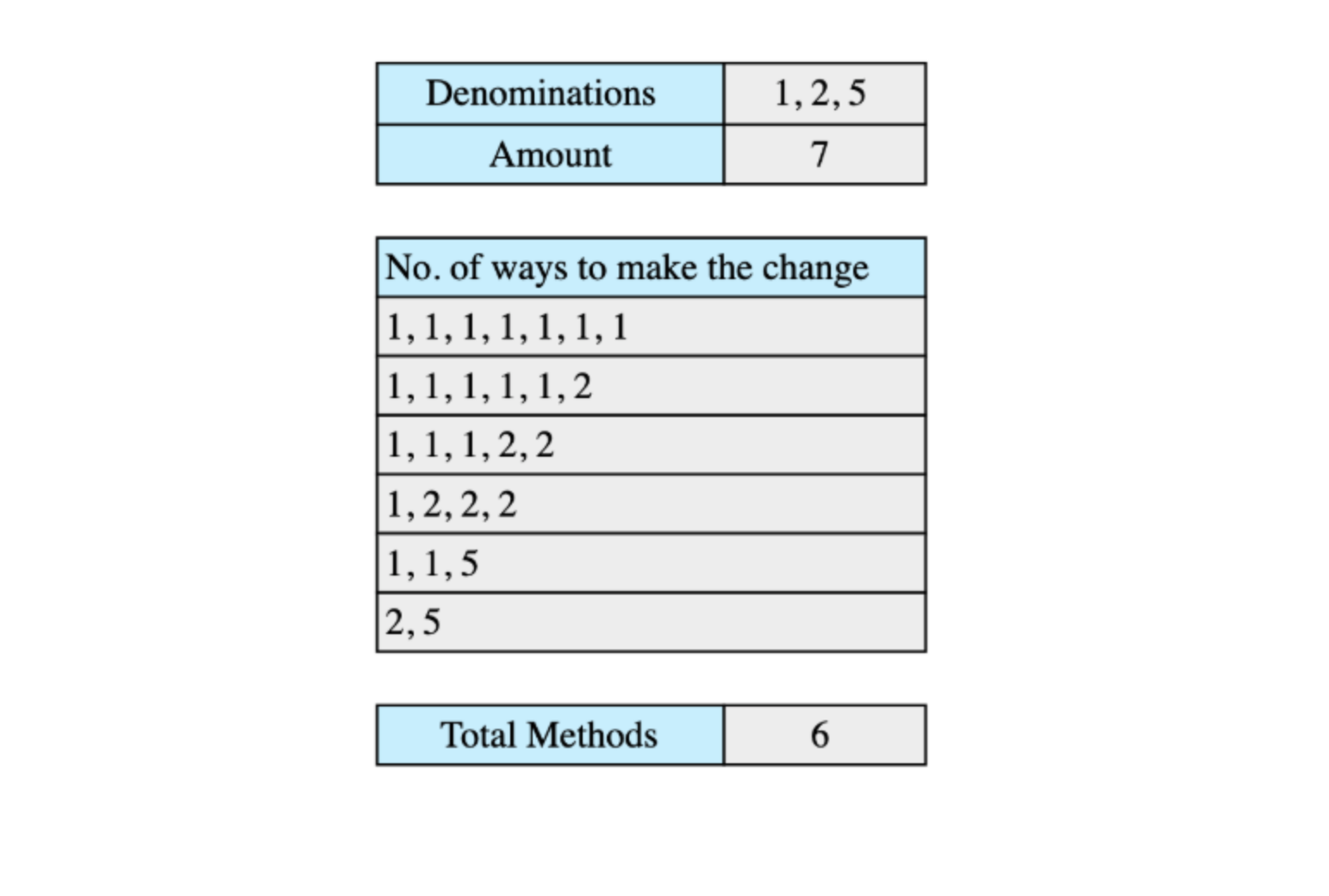def solve_coin_change(denominations, amount):
# TODO: Write - Your - Code
return -1

Click here to view the solution in C++, Java, JavaScript, and Ruby.

Runtime Complexity: Quadratic, $O(m*n)$

Memory Complexity: Linear, $O(n)$

To solve this problem, we’ll keep an array of size amount + 1. One additional space is reserved because we also want to store the solution for the 0 amount.

There is only one way you can make a change of 0, i.e., select no coin so we’ll initialize solution = 1. We’ll solve the problem for each amount, denomination to amount, using coins up to a denomination, den.

The results of different denominations should be stored in the array solution. The solution for amount x using a denomination den will then be:

solution[x] = solution[x] + solution[x - den]


We’ll repeat this process for all the denominations, and at the last element of the solution array, we will have the solution.

### 10. Find Kth permutation

Given a set of ‘n’ elements, find their Kth permutation. Consider the following set of elements:

All permutations of the above elements are (with ordering):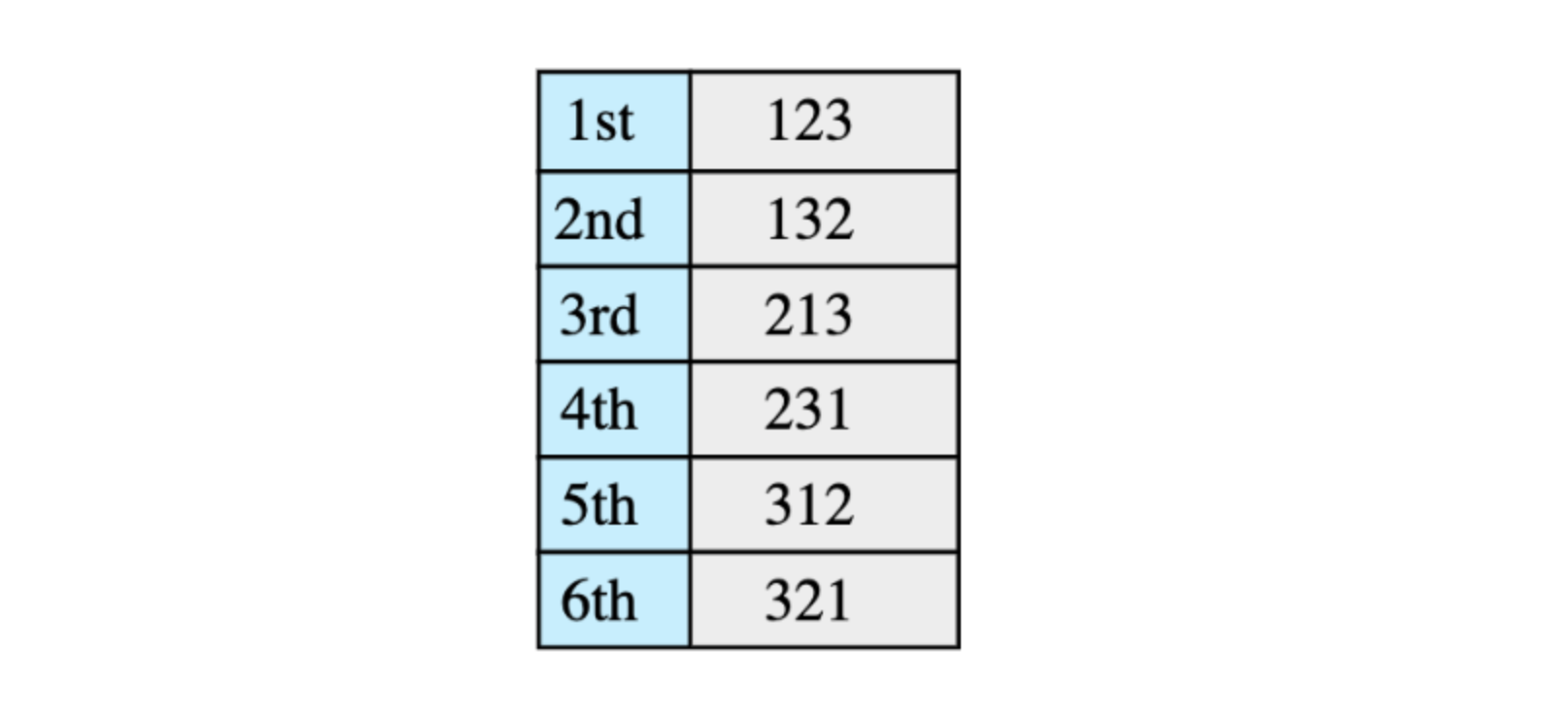Here we need to find the Kth permutation.

def find_kth_permutation(v, k, result):
#TODO: Write - Your - Code
return result

Click here to view the solution in C++, Java, JavaScript, and Ruby.

Runtime Complexity: Linear, $O(n)$

Memory Complexity: Linear, $O(n)$

Here is the algorithm that we will follow:

If input vector is empty return result vector

block_size = (n-1)! ['n' is the size of vector]

Figure out which block k will lie in and select the first element of that block
(this can be done by doing (k-1)/block_size)

Append selected element to result vector and remove it from original input vector

Deduce from k the blocks that are skipped i.e k = k - selected*block_size and goto step 1


#### Succeed in your Amazon interview.

Stop grinding through endless practice questions, and start breaking down 100+ real-world problems. Practice as you learn with hands-on coding environments inside your browser. No need to switch to your IDE, download an SDK, or scrub through videos.

Decode the Coding Interview: Real-World Examples

### 11. Find all subsets of a given set of integers

We are given a set of integers and we have to find all the possible subsets of this set of integers. The following example elaborates on this further.

Given set of integers:

All possile subsets for the given set of integers:

def get_all_subsets(v, sets):
#TODO: Write - Your - Code
return sets

Click here to view the solution in C++, Java, JavaScript, and Ruby.

Runtime Complexity: Exponential, $O(2^n*n)$

Memory Complexity: Exponential, $O(2^n*n)$

There are several ways to solve this problem. We will discuss the one that is neat and easier to understand. We know that for a set of n elements there are $2^n$ subsets. For example, a set with 3 elements will have 8 subsets. Here is the algorithm we will use:

n = size of given integer set
subsets_count = 2^n
for i = 0 to subsets_count
form a subset using the value of 'i' as following:
bits in number 'i' represent index of elements to choose from original set,
if a specific bit is 1 choose that number from original set and add it to current subset,
e.g. if i = 6 i.e 110 in binary means that 1st and 2nd elements in original array need to be picked.
add current subset to list of all subsets



### 12. Print balanced brace combinations

Print all braces combinations for a given value n so that they are balanced. For this solution, we will be using recursion.

def print_all_braces(n):
#TODO: Write - Your - Code
result = []
return result

Click here to view the solution in C++, Java, JavaScript, and Ruby.

Runtime Complexity: Exponential, $2^n$

Memory Complexity: Linear, $O(n)$

The solution is to maintain counts of left_braces and right_braces. The basic algorithm is as follows:​

left_braces count: 0
right_braces count: 0

if left_braces count is less than n:
if right_braces count is less than left_braces count:
stop recursing when left_braces and right_braces counts are both equal to n



### 13. Clone a Directed Graph

Given the root node of a directed graph, clone this graph by creating its deep copy so that the cloned graph has the same vertices and edges as the original graph.

Let’s look at the below graphs as an example. If the input graph is $G = (V, E)$ where V is set of vertices and E is set of edges, then the output graph (cloned graph) G’ = (V’, E’) such that V = V’ and E = E’. We are assuming that all vertices are reachable from the root vertex, i.e. we have a connected graph.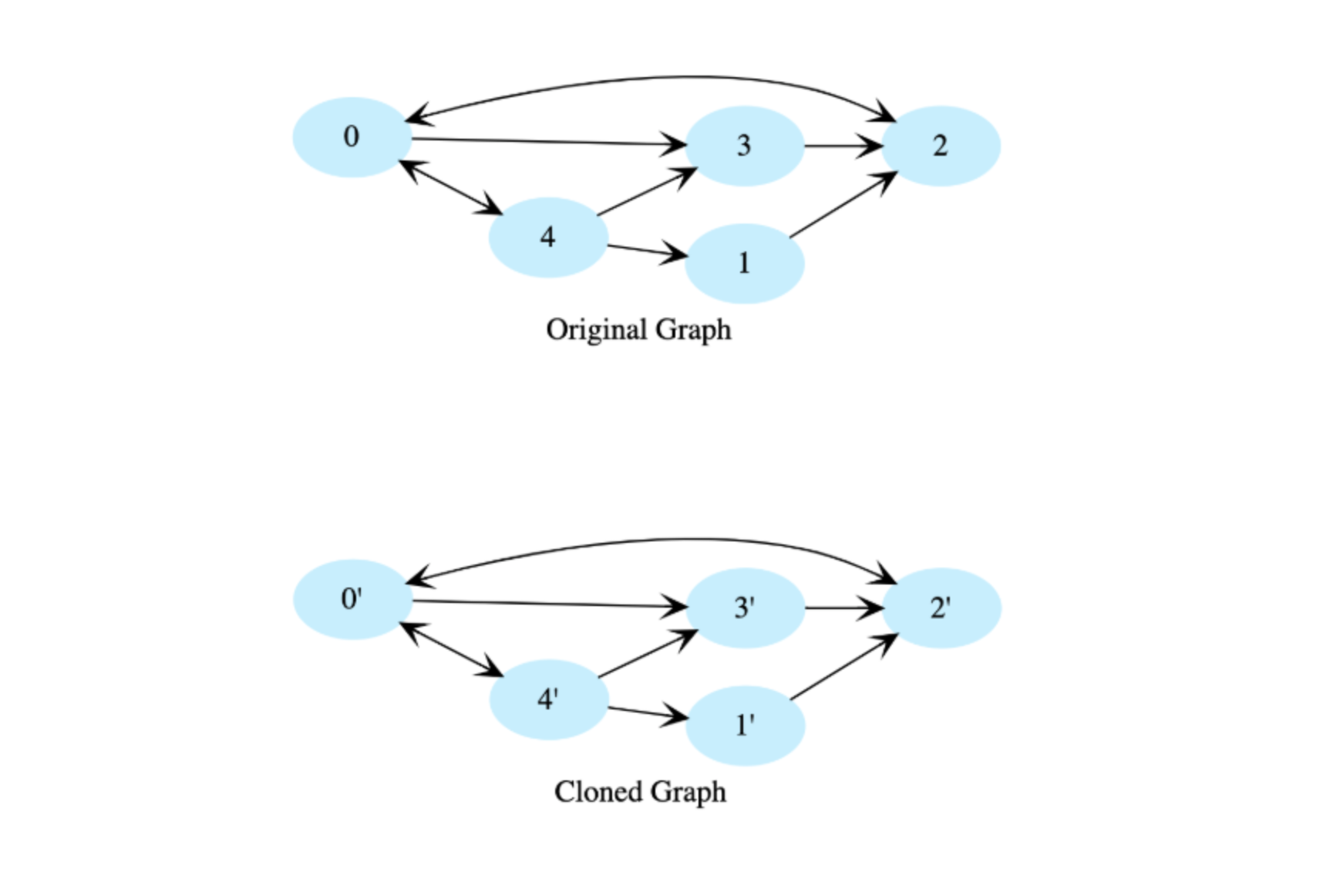class Node:
def __init__(self, d):
self.data = d
self.neighbors = []

def clone(root):
return None    # return root

Click here to view the solution in C++, Java, JavaScript, and Ruby.

Runtime Complexity: Linear, $O(n)$

Memory Complexity: Logarithmic, $O(logn)$

We use depth-first traversal and create a copy of each node while traversing the graph. To avoid getting stuck in cycles, we’ll use a hashtable to store each completed node and will not revisit nodes that exist in the hashtable. The hashtable key will be a node in the original graph, and its value will be the corresponding node in the cloned graph.

### 14. Find Low/High Index

Given a sorted array of integers, return the low and high index of the given key. You must return -1 if the indexes are not found. The array length can be in the millions with many duplicates.

In the following example, according to the key, the low and high indices would be:

• key: 1, low = 0 and high = 0
• key: 2, low = 1 and high = 1
• key: 5, low = 2 and high = 9
• key: 20, low = 10 and high = 10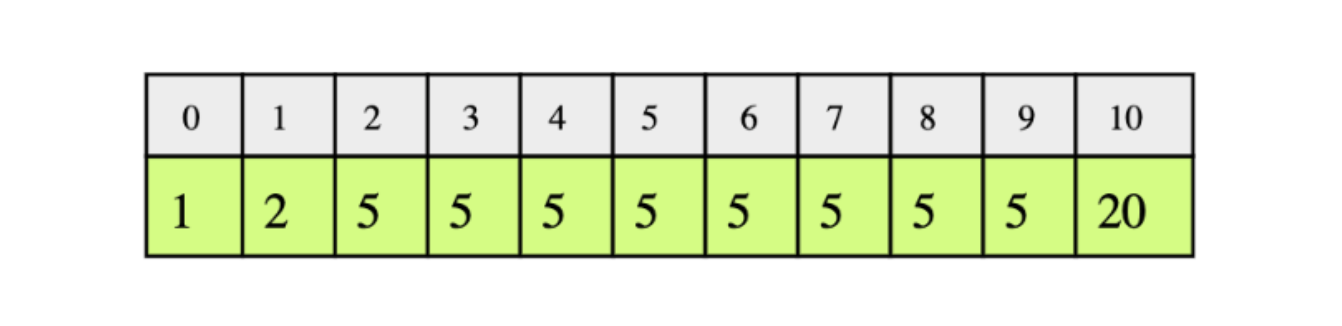For the testing of your code, the input array will be:

1, 1, 1, 2, 2, 2, 2, 2, 3, 3, 3, 4, 4, 4, 4, 5, 5, 5, 6, 6, 6, 6, 6, 6

def find_low_index(arr, key):
#TODO: Write - Your - Code
return -2

def find_high_index(arr, key):
#TODO: Write - Your - Code
return -2

Click here to view the solution in C++, Java, JavaScript, and Ruby.

Runtime Complexity: Logarithmic, $O(logn)$

Memory Complexity: Constant, $O(1)$

Linearly scanning the sorted array for low and high indices are highly inefficient since our array size can be in millions. Instead, we will use a slightly modified binary search to find the low and high indices of a given key. We need to do binary search twice: once for finding the low index, once for finding the high index.

Let’s look at the algorithm for finding the low index. At every step, consider the array between low and high indices and calculate the mid index.

• If the element at mid index is less than the key, low becomes mid + 1 (to move towards the start of range).
• If the element at mid is greater or equal to the key, the high becomes mid - 1. Index at low remains the same.
• When low is greater than high, low would be pointing to the first occurrence of the key.
• If the element at low does not match the key, return -1.

Similarly, we can find the high index by slightly modifying the above condition:

• Switch the low index to mid + 1 when element at mid index is less than or equal to the key.
• Switch the high index to mid - 1 when the element at mid is greater than the key.

### 15. Search Rotated Array

Search for a given number in a sorted array, with unique elements, that has been rotated by some arbitrary number. Return -1 if the number does not exist. Assume that the array does not contain duplicates.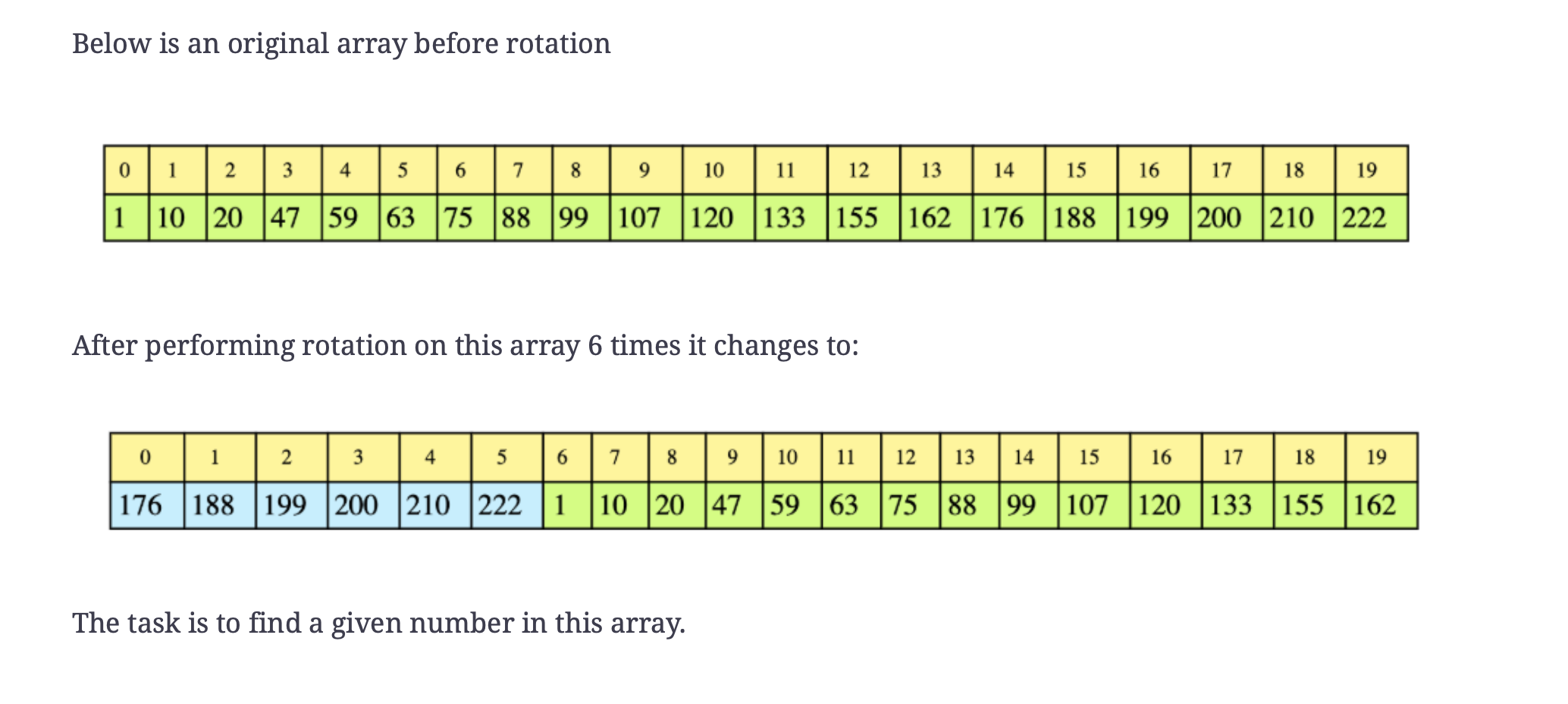def binary_search_rotated(arr, key):
#TODO: Write - Your - Code
return -1

Click here to view the solution in C++, Java, JavaScript, and Ruby.

Runtime Complexity: Logarithmic, $O(logn)$

Memory Complexity: Logarithmic, $O(logn)$

The solution is essentially a binary search but with some modifications. If we look at the array in the example closely, we notice that at least one half of the array is always sorted. We can use this property to our advantage. If the number n lies within the sorted half of the array, then our problem is a basic binary search. Otherwise, discard the sorted half and keep examining the unsorted half. Since we are partitioning the array in half at each step, this gives us $O(log n)$ runtime complexity.

### More common Amazon coding interview questions

1. K largest elements from an array
2. Convert a Binary tree to DLL
3. Given a binary tree T, find the maximum path sum. The path may start and end at any node in the tree.
4. Rotate a matrix by 90 degrees
5. Assembly line scheduling with dynamic programming
6. Implement a stack with push(), min(), and pop() in $O(1)$ time
7. How do you rotate an array by K?
8. Design Snake Game using Object Oriented analysis and design technique.
9. Print all permutations of a given string using recursion
10. Implement a queue using a linked list
11. Find the longest increasing subsequence of an array
12. Lowest common ancestor in a Binary Search Tree and Binary Tree
1. Rotate a given list to the right by k places, which is non-negative.
2. Write a function that counts the total of set bits in a 32-bit integer.
3. How do you detect a loop in a singly linked list?
4. Reverse an array in groups
5. Given a binary tree, check if it’s a mirror of itself
6. Josephus problem for recursion
7. Zero Sum Subarrays
8. Huffman Decoding for greedy algorithms
9. Egg Dropping Puzzle for dynamic programming
10. N-Queen Problem
11. Check if strings are rotations of each other
12. 0-1 Knapsack Problem
13. Unbounded knapsack problem
14. Longest palindromic subsequence
15. Print nth number in the Fibonacci series
16. Longest common substring
17. Longest common subsequence## Overview of Amazon coding interview

To land a software engineering job at Amazon, you need to know what lies ahead. The more prepared you are, the more confident you will be. So, let’s break it down.

• Interview Timeline: The whole interview process takes 6 to 8 weeks to complete.

• Types of Interviews: Amazon coding interviews consist of 5 to 7 interviews. This includes 1 assessment for fit and aptitude, 1-2 online tests, and 4-6 on-site interviews, also known as The Loop.

• The Loop: The onsite interviews include 1 to 3 interviews with hiring managers and 1 bar raiser interview to evaluate Amazon’s 14 leadership principles.

• Coding Questions: Amazon programming questions focus on algorithms, data structures, puzzles, and more.

• Hiring Levels: Amazon usually hires at entry-level 4 (out of 12 total), and the average salary for that level ranges from $106,000 to$114,000 yearly.

• Hiring Teams: Amazon hires based on teams. The most common hiring teams are Alexa and AWS.

• Programming Languages: Amazon prefers the following programming languages for coding questions: Java, C++, Python, Ruby, and Perl.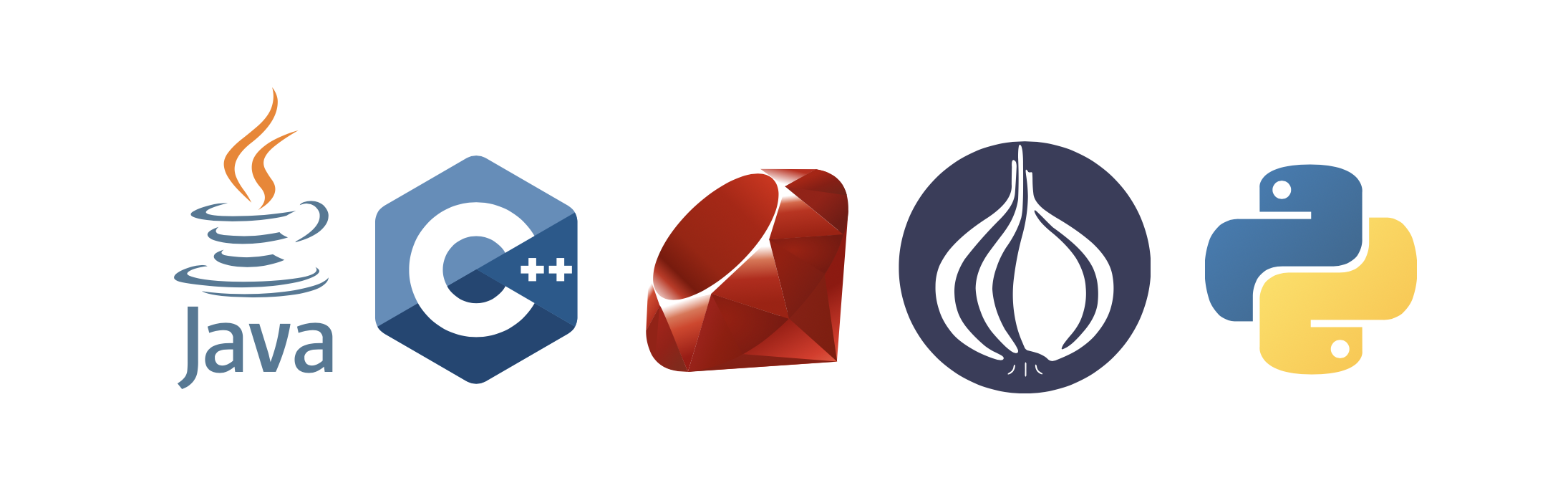Though coding interviews at Amazon are similar to other big tech companies, there are a few differences in their process, in particular, the Bar Raiser. Amazon brings in an objective third-party interviewer called a Bar Raiser, who evaluates candidates on Amazon’s 14 Leadership Principles. The Bar Raiser has complete veto power over whether or not you will be hired.

It is unlikely that you will know which of your interviewers is the Bar Raiser. The key is to take every part of the interview seriously and always assume you’re being evaluated for cultural fit as well as technical competency.

Below are the 14 values that you will be evaluated on.

• Customer Obsession: Leaders start with the customer and work backwards. They work vigorously to earn and keep customer trust.
• Ownership: Leaders are owners. They think long term and don’t sacrifice long-term value for short-term results. They act on behalf of the entire company. They never say “that’s not my job."
• Invent and Simplify: Leaders expect and require innovation and invention from their teams and always find ways to simplify. They are externally aware, look for new ideas from everywhere, and are not limited by “not invented here."
• Are Right, A Lot: Leaders are right a lot. They have strong judgment and good instincts. They seek diverse perspectives and work to disconfirm their beliefs.
• Learn and Be Curious: Leaders are never done learning and always seek to improve themselves.
• Hire and Develop the Best: Leaders raise the performance bar with every hire and promotion. They recognize exceptional talent, and willingly move them throughout the organization.
• Insist on the Highest Standards: Leaders have relentlessly high standards — many people may think these standards are unreasonably high. Leaders are continually raising the bar.
• Think Big: Thinking small is a self-fulfilling prophecy. Leaders create and communicate a bold direction that inspires results.
• Bias for Action: Speed matters in business. Many decisions and actions are reversible and do not need extensive study. We value calculated risk taking.
• Frugality: Accomplish more with less. Constraints breed resourcefulness, self-sufficiency, and invention.
• Earn Trust: Leaders listen attentively, speak candidly, and treat others respectfully. They are vocally self-critical, even when doing so is awkward or embarrassing.
• Dive Deep: Leaders operate at all levels, stay connected to the details, audit frequently, and are skeptical when metrics differ. No task is beneath them.
• Have Backbone; Disagree and Commit: Leaders are obligated to respectfully challenge decisions when they disagree, even when doing so is uncomfortable or exhausting.
• Deliver Results: Leaders focus on the key inputs for their business and deliver them with the right quality and in a timely fashion. Despite setbacks, they rise to the occasion and never settle.
•## How to prepare for an Amazon coding interview

Now that you have a sense of what to expect from an interview and know what kinds of questions to expect, let’s learn some preparation strategies based on Amazon’s unique interview process.

Make sure you’ve updated your resume and LinkedIn profile. Always use deliverables and metrics when you can as they are concrete examples of what you’ve accomplished. Typically recruiters will browse LinkedIn for candidates.

If an Amazon recruiter believes that you are a good match they will reach out to you (via email or LinkedIn) to set up a time to chat. Even if you don’t land the job this time, chatting with them is a great chance to network

### Prepare for coding assessment

It’s up to you to come to a coding interview fully prepared for technical assessment. I recommend at least three months of self-study to be successful. This includes choosing a programming language, reviewing the basics, and studying algorithms, data structures, system design, object-oriented programming, OS, and concurrency concepts.

You’ll also want to prepare for behavioral interviews.

It’s best to find or create an Interview Prep Roadmap to keep yourself on track.

### Prescreen with a recruiter

Expect a light 15-30 minute call where the recruiter will gauge your interest level and determine if you’re a good fit. The recruiter may touch on a few technical aspects. They just want to get an idea of your skills. Typical questions might include your past work experiences, your knowledge of the company/position, salary, and other logistical questions.

It’s important to have around 7-10 of your own questions ready to ask the interviewer. Asking questions at an early stage shows investment and interest in the role.

### Online Assessment

Once you complete the call with the recruiter, they’ll administer an online coding test, a debugging test, and an aptitude test. The debugging section will have around 6-7 questions, which you have 30 minutes to solve. The aptitude section will have around 14 multiple-choice questions, dealing with concepts like basic permutation combination and probabilities.

The coding test consists of two questions. You’ll have about 1.5 hours to complete it. Expect the test to be conducted through Codility, HackerRank, or another site. Expect some easy to medium questions that are typically algorithm related. Examples include: ​

• Reverse the second half of a linked list ​
• Find all anagrams in a string ​
• Merge overlapping intervals

### Phone Interviews

Once you’ve made it past the prescreen and online assessment, the recruiter will schedule your video/phone interviews, likely with a hiring manager or a manager from the team you’re looking to join. At this stage in the process, there will be one to three more interviews.

This is where they’ll ask you questions directly related to your resume, as well as data structures, algorithms, and other various coding questions that apply to the position. You can expect to write code, review code, and demonstrate your technical knowledge.

### On-Site Interviews: The Loop

If you have successfully made it through the series of phone interviews, you’ll be invited for an on-site visit. This full day of on-site interviews is referred to as the “The Loop”. Throughout the day, you’ll meet with 4-6 people. Expect half of these interviews to be technical and the other half to assess soft skills. Be prepared to work through questions on a whiteboard and discuss your thought process.

Concepts that Amazon loves to test on are data structures and algorithms. It’s important to know the runtimes, theoretical limitations, and basic implementation strategies of different classes of algorithms.

• Data structures you should know: Arrays, Stacks, Queues, Linked lists, Trees, Graphs, Hash tables

• Algorithms you should know: Breadth First Search, Depth First Search, Binary Search, Quicksort, Mergesort, Dynamic programming, Divide and Conquer

### The Offer / No Offer

Generally, you’ll hear back from a recruiter within a week after your interviews. If you didn’t get an offer, Amazon will give you a call. You’ll likely have to wait another six months to re-apply. Judging that your on-site interviews went well, they’ll reach out to you, at which point they’ll make you an offer, send you documents to sign, and discuss any further questions you have.

## Wrapping up and resources

Cracking the Amazon coding interview comes down to the time you spend preparing, such as practicing coding questions, studying behavioral interviews, and understanding Amazon’s company culture. There is no golden ticket, but more preparation will surely make you a more confident and desirable candidate.

To help you prepare for interviews, Educative has created the unique course Decode the Coding Interview: Real-World Examples. Available in multiple languages, this course takes you through 100+ real-world questions like these. After each project, you’ll better understand what techniques you just applied.

This is coding interview prep reimagined, with your needs in mind.

Happy learning